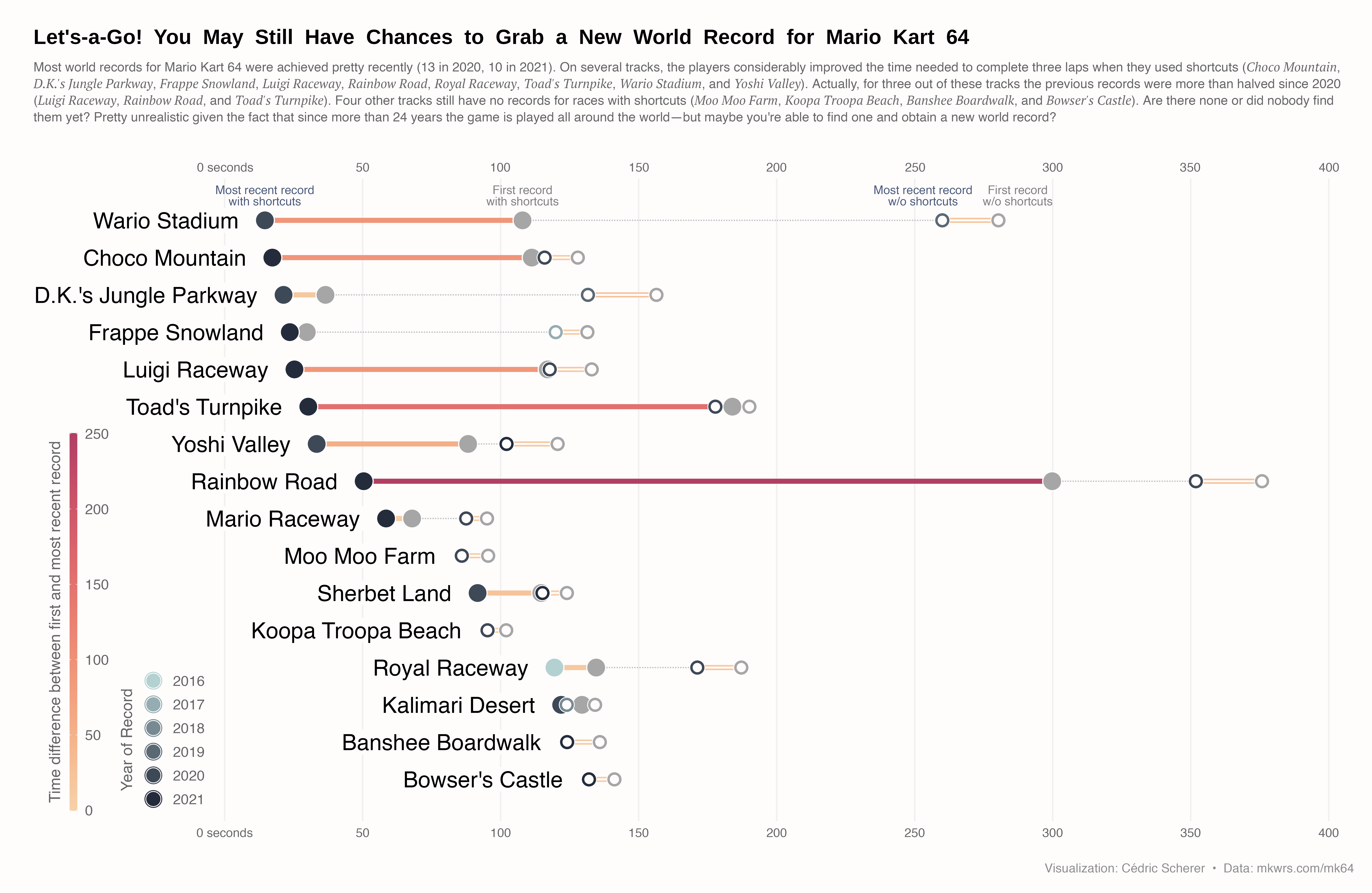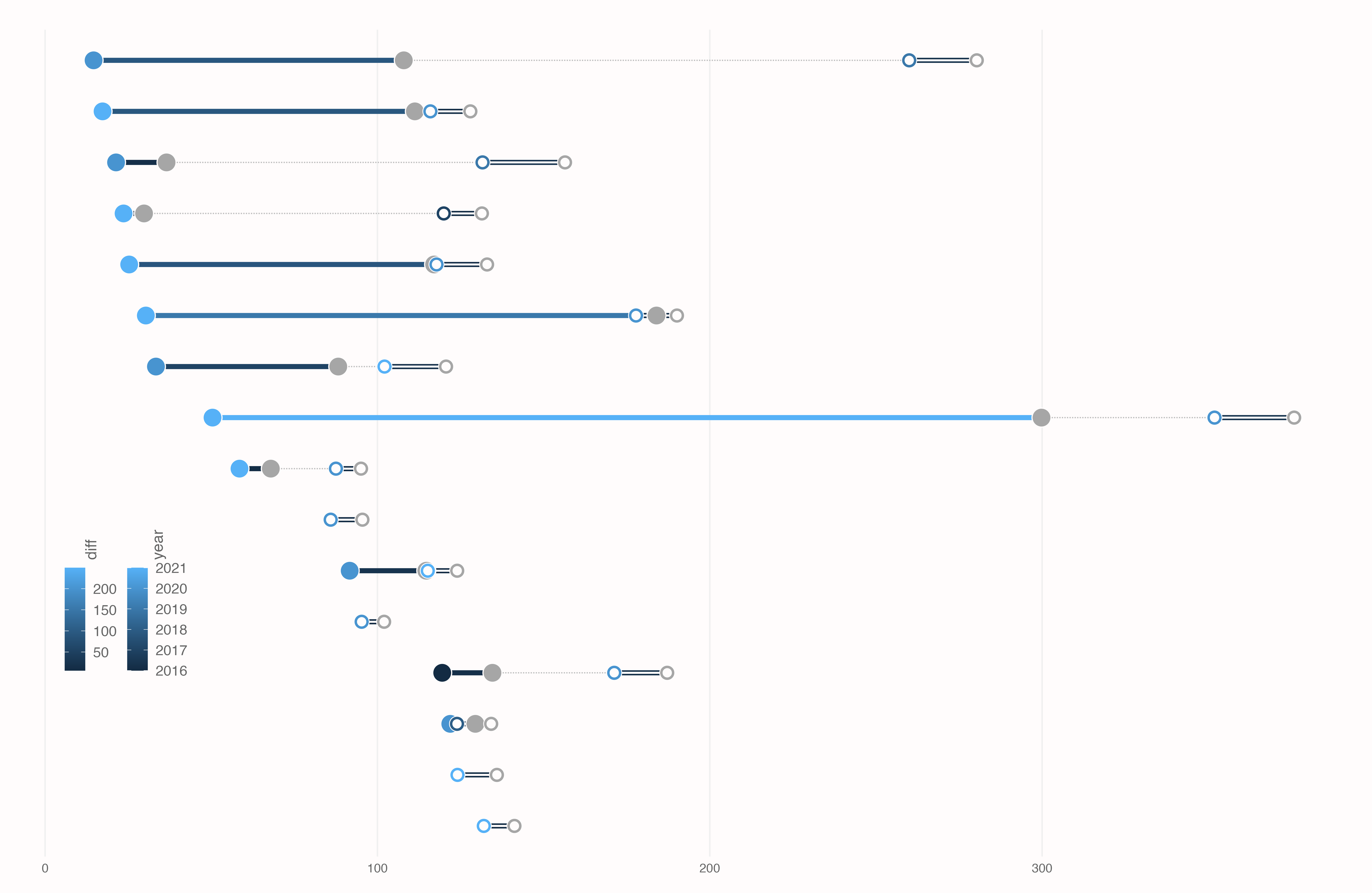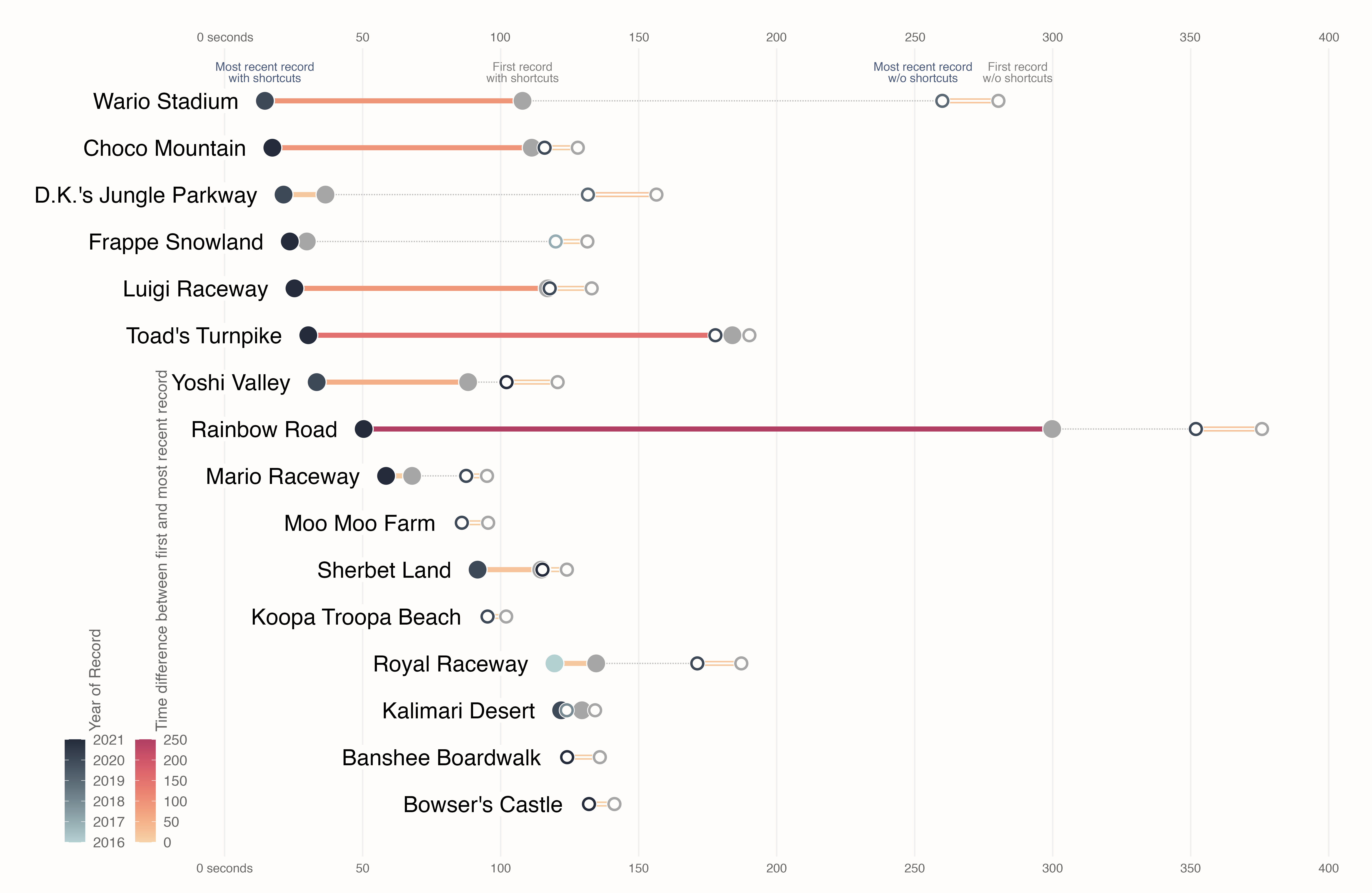# Mario Kart 64 World Records

A highly customized lollipop chart showing world records for the Mario Kart 64 racing game on the Nintendo 64. Built with `R` and the `tidyverse`. This article shows how to use more than one data set in a `ggplot2` chart and how to save plots with the `ragg` package.

This page showcases the work of Cedric Scherer, built for the TidyTuesday initiative. You can find the original code on his github repository here.

Thanks to him for accepting sharing his work here! 🙏🙏 Thanks also to Tomás Capretto who help writing down the blogpost!

As a teaser, here is the plot we’re gonna try building:## Package and Theme

Let’s start by loading the packages needed to build the figure. From all the packages we use, ragg may be the least known. It’s a great library that impacts both the quality and performance of our graphs in `R` thanks to the new graphic devices it brings through the lower-level AGG library.

``````# Load packages
library(tidyverse)
library(ggtext)
library(ragg)

# Set ggplot theme
theme_set(theme_minimal(base_family = "Atlantis", base_size = 13))
theme_update(
plot.margin = margin(25, 15, 15, 25),
plot.background = element_rect(color = "#FFFCFC", fill = "#FFFCFC"),
panel.grid.major.x = element_line(color = "grey94"),
panel.grid.major.y = element_blank(),
panel.grid.minor = element_blank(),
axis.text = element_text(family = "Hydrophilia Iced"),
axis.text.x = element_text(color = "grey40"),
axis.text.y = element_blank(),
axis.title = element_blank(),
axis.ticks = element_blank(),
legend.position = c(.07, .31),
legend.title = element_text(
color = "grey40",
family = "Overpass",
angle = 90,
hjust = .5
),
legend.text = element_text(
color = "grey40",
family = "Hydrophilia Iced",
size = 12
),
legend.box = "horizontal",
legend.box.just = "bottom",
legend.margin = margin(0, 0, 0, 0),
legend.spacing = unit(.6, "lines"),
plot.title = element_text(
face = "bold",
size = 17.45
),
plot.subtitle = element_textbox_simple(
family = "Overpass",
color = "grey40",
size = 10.8,
lineheight = 1.3,
margin = margin(t = 5, b = 30)
),
plot.caption = element_text(
family = "Overpass",
color = "grey55",
size = 10.5,
margin = margin(t = 20, b = 0, r = 15)
)
)``````

# Load and prepare the dataset

The dataset provides world records for the Mario Kart 64 game. The game consists of 16 individual tracks and world records can be achieved for the fastest single lap or the fastest completed race (three laps). Also, through the years, players discovered shortcuts in many of the tracks. Fortunately, shortcut and non-shortcut world records are listed separately.

Our chart consists of a double-dumbbell plot, a variation of the better known lollipop chart, where we visualize world record times on Mario Kart 64 with and without shortcuts. The original source of the data is available here. It holds time trial world records for all of the Mario Kart games, but we are using the version released for the TidyTuesday initiative on the week of 2021-05-25. You can find the original announcement and more information about the data here.

``df_records <- readr::read_csv('https://raw.githubusercontent.com/rfordatascience/tidytuesday/master/data/2021/2021-05-25/records.csv')``

From all the columns in the data, we only use `track`, `type`, `shortcut`, `date`, and `time`.

• `track` indicates the name of the track
• `type` tells us whether the record is for single lap or a complete race
• `shortcut` is a yes/no variable that identifies records where a shortcut was used
• `date` represents the date where the record was achieved
• `time` indicates how many seconds it took to complete the track.

On this occasion, we only keep records for complete races. We start by creating `df_rank`, a data frame that keeps current world records for every track. The two last `arrange()` and `mutate()` lines are used to convert `track` into an ordered factor where the order is given by the time it took to complete the race. It will be useful when sorting tracks on the plot.

``````df_rank <-
df_records %>%
filter(type == "Three Lap") %>%
group_by(track) %>%
filter(time == min(time)) %>%
ungroup %>%
arrange(-time) %>%
mutate(track = fct_inorder(track, time))``````

We also create `df_records_three` which holds all the records, no matter they were beaten or not. It is used to derive other data frames that are used in our chart.

``````df_records_three <-
df_records %>%
filter(type == "Three Lap") %>%
mutate(year = lubridate::year(date)) %>%
mutate(track = factor(track, levels = levels(df_rank\$track)))``````

The first data frame derived is `df_connect`. This one is used to add a dotted line that connects record times with and without shortcuts.

``````df_connect <-
df_records_three %>%
group_by(track, type, shortcut) %>%
summarize(no = min(time), yes = max(time)) %>%
pivot_longer(
cols = -c(track, type, shortcut),
names_to = "record",
values_to = "time"
) %>%
# The line goes from the first record without shortcut (the slower)
# to the most recent record with shortcut (the fastest)
filter((shortcut == "No" & record == "no") | (shortcut == "Yes" & record == "yes")) %>%
pivot_wider(id_cols = c(track), values_from = time, names_from = record)``````

Then we have `df_longdist` and `df_shortcut`. Without getting much into the details, we note each data frame consists of five columns: `track`, `year`, `max`, `min` and `diff`. `year` refers to the year where the current record was achieved, `max` is the completetion time for the first record and `min` is the time for the current record. `diff` is simply the difference between `max` and `min`, i.e. a measurement of how much the first record was improved. `df_shortcut` and `df_longdist` refer to records with and without shortcuts, respectively.

``````df_longdist <-
df_records_three %>%
filter(shortcut == "No") %>%
group_by(track) %>%
filter(time == min(time) | time == max(time)) %>%
mutate(group = if_else(time == min(time), "min", "max")) %>%
group_by(track, group) %>%
arrange(time) %>%
slice(1) %>%
group_by(track) %>%
mutate(year = max(year)) %>%
pivot_wider(id_cols = c(track, year), values_from = time, names_from = group) %>%
mutate(diff = max - min)

df_shortcut <-
df_records_three %>%
filter(shortcut == "Yes") %>%
group_by(track) %>%
filter(time == min(time) | time == max(time)) %>%
mutate(group = if_else(time == min(time), "min", "max")) %>%
group_by(track, group) %>%
arrange(time) %>%
slice(1) %>%
group_by(track) %>%
mutate(year = max(year)) %>%
pivot_wider(id_cols = c(track, year), values_from = time, names_from = group) %>%
mutate(diff = max - min)``````

## Basic Plot

In this case, our lollipop plot consists of several overlapped geoms. As always there’s intentional overlap in a plot, we add what we want to remain on the background first.

``````# This dataset `df_shorcut` and the aesthetics `aes(min, track)` are used
# in the `geom_*` calls when they are not explicitly specified.
p <- df_shortcut %>%
ggplot(aes(min, track)) +
# Dotted line connection shortcut yes/no
# This geom uses `df_connect` instead of `df_shorcut` because it is being
# explicitly overridden
geom_linerange(
data = df_connect,
aes(xmin = yes, xmax = no, y = track),
inherit.aes = FALSE,
color = "grey75",
linetype = "11" # dotted line
) +
# Segment when shortcut==yes
# When the `data` argument is missing in the `geom_*` function
geom_linerange(aes(xmin = min, xmax = max, color = diff), size = 2) +
# Segment when shortcut==no. Overlapped lineranges.
geom_linerange(data = df_longdist, aes(xmin = min, xmax = max, color = diff), size = 2) +
geom_linerange(data = df_longdist, aes(xmin = min, xmax = max), color = "#FFFCFC", size = .8)``````While there’s a lot we still have to customize yet, this preview lets us see that the white center in the lines is used to differentiate records without shortcuts from those records with shortcuts.

## Customized lollipop Plot

The same logic is used in the next chunk to add dots representing the first and last world records for each modality.

``````p <- p +
# Point when shortcut==yes – first record
geom_point(aes(x = max), size = 7, color = "#FFFCFC", fill = "grey65", shape = 21, stroke = .7) +
# Point when shortcut==yes – latest record.
geom_point(aes(fill = year), size = 7, color = "#FFFCFC", shape = 21, stroke = .7) +
# Point when shortcut==no – first record.
geom_point(data = df_longdist, aes(fill = year), size = 5.6, shape = 21,
color = "#FFFCFC", stroke = .5) +
geom_point(data = df_longdist, size = 3, color = "#FFFCFC") +
# Point when shortcut==no – latest record
geom_point(data = df_longdist, aes(x = max), size = 5.6, shape = 21,
fill = "grey65", color = "#FFFCFC", stroke = .5) +
geom_point(data = df_longdist, aes(x = max), size = 3, color = "#FFFCFC")``````And it is now clear that records without shortcuts are represented by geoms with white centers.

Let’s keep improving the appearence of the chart. Now it’s the turn to customize labels and legends, and add some informative text.

First, add the track labels with `geom_label()`. We need to make two calls because some tracks don’t have any record without shortcuts and so their names are taken from the `df_longdist` data frame. Next, `geom_text()` is used four times to label the four different types of dots and tell the reader what they meaning. This is a very nice approach to include information on a plot without a legend.

``````p <- p +
## labels tracks
geom_label(aes(label = track), family = "Atlantis", size = 6.6, hjust = 1, nudge_x = -7,
label.size = 0, fill = "#FFFCFC") +
geom_label(data = filter(df_longdist, !track %in% unique(df_shortcut\$track)),
aes(label = track), family = "Atlantis", size = 6.6, hjust = 1, nudge_x = -7,
label.size = 0, fill = "#FFFCFC") +
## labels dots when shortcut==yes
geom_text(data = filter(df_shortcut, track == "Wario Stadium"),
aes(label = "Most recent record\nwith shortcuts"),
family = "Overpass", size = 3.5, color = "#4a5a7b",
lineheight = .8, vjust = 0, nudge_y = .4) +
geom_text(data = filter(df_shortcut, track == "Wario Stadium"),
aes(x = max, label = "First record\nwith shortcuts"),
family = "Overpass", size = 3.5, color = "grey50",
lineheight = .8, vjust = 0, nudge_y = .4) +
## labels dots when shortcut==no
geom_text(data = filter(df_longdist, track == "Wario Stadium"),
aes(label = "Most recent record\nw/o shortcuts"),
family = "Overpass", size = 3.5, color = "#4a5a7b", lineheight = .8,
vjust = 0, nudge_x = -7, nudge_y = .4) +
geom_text(data = filter(df_longdist, track == "Wario Stadium"),
aes(x = max, label = "First record\nw/o shortcuts"),
family = "Overpass", size = 3.5, color = "grey50", lineheight = .8,
vjust = 0, nudge_x = 7, nudge_y = .4)``````

Then it’s the time to tweak scales and axis.

``````p <- p +
# Extend horizontal axis so trackl labels fit
coord_cartesian(xlim = c(-60, 400)) +
scale_x_continuous(
breaks = seq(0, 400, by = 50),
# Add 'seconds' label only to first axis tick
labels = function(x) ifelse(x == 0, paste(x, "seconds"), paste(x)),
sec.axis = dup_axis(), # Add axis ticks and labels both on top and bottom.
expand = c(.02, .02)
) +
scale_y_discrete(expand = c(.07, .07)) +
scale_fill_gradient(low = "#b4d1d2", high = "#242c3c", name = "Year of Record") +
rcartocolor::scale_color_carto_c(
palette = "RedOr",
limits = c(0, 250),
name = "Time difference between first and most recent record"
)``````Some touches to the position of the guides and their size…

``````p <- p +
guides(
fill = guide_legend(title.position = "left"),
color = guide_colorbar(
barwidth = unit(.45, "lines"),
barheight = unit(22, "lines"),
title.position = "left"
)
) +
labs(
title = "Let's-a-Go!  You  May  Still  Have  Chances  to  Grab  a  New  World  Record  for  Mario  Kart  64",
subtitle = "Most world records for Mario Kart 64 were achieved pretty recently (13 in 2020, 10 in 2021). On several tracks, the players considerably improved the time needed to complete three laps when they used shortcuts (*Choco Mountain*, *D.K.'s Jungle Parkway*, *Frappe Snowland*, *Luigi Raceway*, *Rainbow Road*, *Royal Raceway*, *Toad's Turnpike*, *Wario Stadium*, and *Yoshi Valley*). Actually, for three out of these tracks the previous records were more than halved since 2020 (*Luigi Raceway*, *Rainbow Road*, and *Toad's Turnpike*). Four other tracks still have no records for races with shortcuts (*Moo Moo Farm*, *Koopa Troopa Beach*, *Banshee Boardwalk*, and *Bowser's Castle*). Are there none or did nobody find them yet? Pretty unrealistic given the fact that since more than 24 years the game is played all around the world—but maybe you're able to find one and obtain a new world record?",
caption = "Visualization: Cédric Scherer  •  Data: mkwrs.com/mk64"
)``````And finally, save the plot. `device = agg_png` means we use the PNG graphic device from the AGG backend previously mentioned instead of the default PNG backend that comes with a typical installation of R.

``````ggsave(
here::here("img", "lollipop-plot-with-r-mario-kart-64-world-records.png"),
width = 7990, height = 5200, res = 500, device = agg_png, limitsize = FALSE
)``````

## Conclusion

Here we are, with a very highly customized plot showcasing the possibilities offered by the tidyverse and other packages like `ragg`. Thanks again to Cedric for providing this chart example!

Related chart types

## Contact

This document is a work by Yan Holtz. Any feedback is highly encouraged. You can fill an issue on Github, drop me a message on Twitter, or send an email pasting yan.holtz.data with gmail.com.